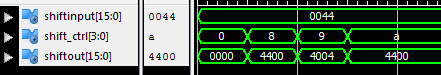## VHDL code for the shifter will be presented together with its testbench VHDL code for functional simulation.#### The input/ output interface of the shifter is shown in the above figure. The shifter instruction set is as follows:

• SHIFT_Ctrl = "1000": SHIFTOUT <= Rotate SHIFTINPUT >>8
• SHIFT_Ctrl = "1001": SHIFTOUT <= Rotate SHIFTINPUT >>4
• SHIFT_Ctrl = "1010": SHIFTOUT <= Shift Left Logical SHIFTINPUT << 8

### VHDL code for the shifter:

```library IEEE;
use IEEE.STD_LOGIC_1164.ALL;
-- fpga4student.com: FPGA projects, Verilog projects, VHDL projects
-- VHDL project: VHDL code for Shifter
entity shifter is
generic ( N: integer:=16
);
Port ( SHIFTINPUT : in  STD_LOGIC_VECTOR(N-1 downto 0);
SHIFT_Ctrl : in  STD_LOGIC_VECTOR(3 downto 0);
SHIFTOUT: out  STD_LOGIC_VECTOR(N-1 downto 0)
);
end shifter;

architecture Behavioral of shifter is

begin
process(SHIFTINPUT,SHIFT_Ctrl)
begin
case(SHIFT_Ctrl) is
when "1000" => SHIFTOUT <= SHIFTINPUT(7 downto 0)&SHIFTINPUT(15 downto 8);-- ROR8
when "1001" => SHIFTOUT <= SHIFTINPUT(3 downto 0)&SHIFTINPUT(15 downto 4);-- ROR4
when "1010" => SHIFTOUT <= SHIFTINPUT(7 downto 0) & "00000000"; -- SLL8
when others => SHIFTOUT <= x"0000";
end case;
end process;

end Behavioral;```

### Testbench VHDL code for the shifter:

```LIBRARY ieee;
USE ieee.std_logic_1164.ALL;
-- fpga4student.com: FPGA projects, Verilog projects, VHDL projects
-- VHDL project: VHDL code for Shifter
-- VHDL testbench code for Shifter
ENTITY tb_shifter IS
END tb_shifter;

ARCHITECTURE behavior OF tb_shifter IS

-- Component Declaration for Shifter
COMPONENT shifter
PORT(
SHIFTINPUT : IN  std_logic_vector(15 downto 0);
SHIFT_Ctrl : IN  std_logic_vector(3 downto 0);
SHIFTOUT : OUT  std_logic_vector(15 downto 0)
);
END COMPONENT;

--Inputs
signal SHIFTINPUT : std_logic_vector(15 downto 0) := (others => '0');
signal SHIFT_Ctrl : std_logic_vector(3 downto 0) := (others => '0');
--Outputs
signal SHIFTOUT : std_logic_vector(15 downto 0);

BEGIN

-- Instantiate the Shifter
uut: shifter PORT MAP (
SHIFTINPUT => SHIFTINPUT,
SHIFT_Ctrl => SHIFT_Ctrl,
SHIFTOUT => SHIFTOUT
);

-- Stimulus process for shifter
stim_proc: process
begin
SHIFTINPUT <= x"0044";
wait for 100 ns;
SHIFT_Ctrl <= "1000";-- ROR8
wait for 100 ns;
SHIFT_Ctrl <= "1001";-- ROR4
wait for 100 ns;
SHIFT_Ctrl <= "1010";-- SLL8
wait;
end process;

END;```

#### Synthesized RTL Schematic for the shifter:#### Simulation Waveform for the shifter:Recommended VHDL projects:
1. What is an FPGA? How VHDL works on FPGA
2. VHDL code for FIFO memory
3. VHDL code for FIR Filter
4. VHDL code for 8-bit Microcontroller
5. VHDL code for Matrix Multiplication
6. VHDL code for Switch Tail Ring Counter
7. VHDL code for digital alarm clock on FPGA
8. VHDL code for 8-bit Comparator
9. How to load a text file into FPGA using VHDL
10. VHDL code for D Flip Flop
11. VHDL code for Full Adder
12. PWM Generator in VHDL with Variable Duty Cycle
13. VHDL code for ALU
14. VHDL code for counters with testbench
15. VHDL code for 16-bit ALU